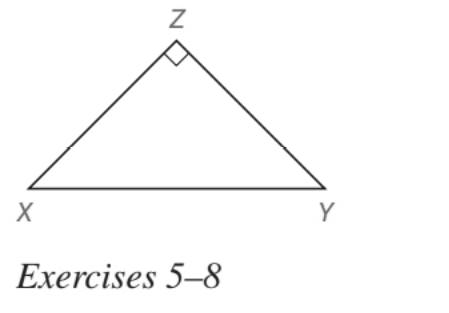Chapter 5.5, Problem 6EElementary Geometry For College St...

7th Edition
Alexander + 2 others
ISBN: 9781337614085

Solutions

Chapter
SectionElementary Geometry For College St...

7th Edition
Alexander + 2 others
ISBN: 9781337614085
Textbook Problem

In Exercises 5 to 22, find the missing lengths. Give your answers in both simplest radical form and as approximations correct two decimal places. Given: Right ∆ X Y Z with X Z ¯ ≅ Y Z ¯ and X Y = 10 Find: X Z and Y ZTo determine

To find:

XZ and YZ for the right XYZ with XZ¯YZ¯ and XY=10.

Explanation

Approach:

For a right triangle for which the measure of the interior angles 45°, 45°, and 90°, if ‘a’ is the length of measure of one of the leg; opposite to the angle 45°, then the length of the other two sides is given by

Length of the other leg =a

Length of the hypotenuse =a2

In general

Length of the legs are equal.

Length of the hypotenuse =2× (Length of one of the legs)

Calculation:

Given,

The right XYZ with XZ¯YZ¯ and XY=10.

Since, two of the sides of the right triangle are congruent, the angles opposite to the sides must be equal and should be 45° each.

Thus, the XYZ become

Here, XY is the hypotenuse of the XYZ.

By the application of 45°-45°-90°

45°-45°-90° theorem.

In a right triangle whose angle measures 45°, 45°, and 90°, the legs are congruent and the hypotenuse has a length equal to the product of 2 and the length of either leg

Still sussing out bartleby?

Check out a sample textbook solution.

See a sample solution

The Solution to Your Study Problems

Bartleby provides explanations to thousands of textbook problems written by our experts, many with advanced degrees!

Get Started

Write the number in polar form with argument between 0 and 2. 27. 3 + 4i

Single Variable Calculus: Early Transcendentals, Volume I

In Exercises 13-20, sketch a set of coordinate axes and plot each point. 14. (1, 3)

Applied Calculus for the Managerial, Life, and Social Sciences: A Brief Approach

Evaluate the integrals in Problems 1-26. 15.

Mathematical Applications for the Management, Life, and Social Sciences

If r=dr,d=1050,andr=21,findt.

Elementary Technical Mathematics

True or False: If a function is not increasing on an interval, then it is decreasing on the interval.

Study Guide for Stewart's Single Variable Calculus: Early Transcendentals, 8th